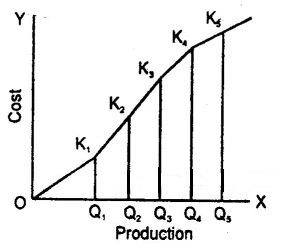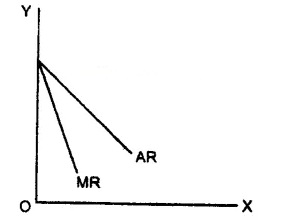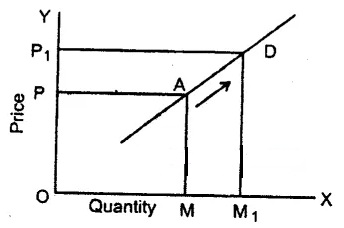Check the below NCERT MCQ Questions for Class 11 Economics Chapter 3 Production and Costs with Answers Pdf free download. MCQ Questions for Class 11 Economics with Answers were prepared based on the latest exam pattern. We have provided Production and Costs Class 11 Economics MCQs Questions with Answers to help students understand the concept very well.

## Production and Costs Class 11 MCQs Questions with Answers

Class 11 Economics Chapter 3 MCQ Question 1.
In production function, production is a function of:
(a) Price
(b) Factors of Production
(c) Total Expenditure
(d) None of these

MCQ Questions For Class 11 Economics Chapter 3 Question 2.
The basic reason of operating the Law of Diminishing Returns is:
(a) Scarcity of Factors
(b) Imperfect Substitution between Factors
(c) Both (a) and (b)
(d) None of the above

Answer: (c) Both (a) and (b)

Chapter 3 Economics Class 11 MCQ Question 3.
Which of the following explains the short-run production function ?
(a) Law of Demand
(b) Law of Variable Proportion
(c) Returns to Scale
(d) Elasticity of Demand

Answer: (b) Law of Variable Proportion

Economics Class 11 Chapter 3 MCQ Question 4.
Long-run production function is related to:
(a) Law of Demand
(b) Law of Increasing Returns
(c) Laws of Returns to Scale
(d) Elasticity of Demand

Answer: (c) Laws of Returns to Scale

Question 5.
In which stage of production a rational producer likes to operate in shot-run production ?
(a) First Stage
(b) Second Stage
(c) Third Stage
(d) None of these

Question 6.
Law of variable proportion explains three stages of production. In the first stage of production:
(a) Both MP and AP rise
(b) MP rises
(c) AP Falls
(d) MP is zero

Answer: (a) Both MP and AP rise

Question 7.
At which time all the factors of production may be changed ?
(a) Short run
(b) Long run
(c) Very Long run
(d) All the three

Question 8.
Production function is expressed as:
(a) Qx = Px
(b) Qx = f(A, B, C, D)
(c) Qx = Dx
(d) None of these

Answer: (b) Qx = f(A, B, C, D)

Question 9.
Which factors among following we find in short-run production process ?
(a) Fixed Factors
(b) Variable Factors
(c) Both (a) and (b)
(d) None of these

Answer: (c) Both (a) and (b)

Question 10.
The cycle which increases first and after being constant starts to reduce is called :
(a) APP
(b) MPP
(c) TPP
(d) All of these

Question 11.
Which of the following is a saurce of production ?
(a) Land
(b) Labour
(c) Capital
(d) All of these

Question 12.
Law of variable proportion is related to :
(a) Both short-run and long run
(b) Long-run
(c) Short-run
(d) Very Long-run

Question 13.
An active factor of production is:
(a) Capital
(b) Labour
(c) Land
(d) None of these

Question 14.
If all the factors of production are increased by same proportion and as a result output increases by a greater proportion than it is called :
(a) Constant returns to scale
(b) Decreasing returns to scale
(d) All of these
(d) None of these

Question 15.
Which of the following is included in money cost ?
(a) Normal Profit
(b) Explicit Cost
(c) Implicit Cost
(d) All of these

Question 16.
Which of the following is not fixed cost ?
(b) Interest
(c) Cost of Raw Material
(d) Rent of the Factory

Answer: (c) Cost of Raw Material

Question 17.
With the increase in production the difference between total cost and total fixed cost:
(a) Remains Constant
(b) Increases
(c) Decreases
(d) Both Increases or Decreases

Question 18.
Changes in production quantity affect:
(a) Both Fixed and Variable Cost
(b) Only Variable Cost
(c) Only Fixed Cost
(d) None of the above

Question 19.
What happens when production is shut down ?
(a) Fixed Cost Increases
(b) Variable Costs Decline
(c) Variable Costs become zero
(d) Fixed Costs become zero

Answer: (c) Variable Costs become zero

Question 20.
The alternative name of opportunity cost is:
(a) Economic Cost
(b) Equilibrium Price
(c) Marginal Cost
(d) Average Cost

Question 21.
When average cost is decreasing what status marginal cost has as compared to average cost ?
(a) MC > AC
(b) MC = AC
(c) MC ≤ AC
(d) MC ≠ AC

Question 22.
Which statement of the following is true ?
(a) AC=TFC – TVC
(b) AC = AFC + TVC
(c) AC=TFC + AVC
(d) AC = AFC + AVC

Answer: (d) AC = AFC + AVC

Question 23.
What is an opportunity cost ?
(a) The alternative foregon
(b) The opportunity lost
(c) Transfer earnings
(d) All of these

Question 24.
The shape of average cost curve is :
(a) U-shaped
(b) Reactangular Hyperbola shaped
(c) Line parallel to x-axis
(d) None of these

Question 25.
The average fixed cost at 5 units of output is Rs. 20. Average variable cost at 5 units of output is Rs. 40. Average cost of producing 5 units is:
(a) Rs. 20
(b) Rs. 40
(c) Rs.56
(d) Rs.60

Question 26.
Which of the following is correct ?
(a) TVC = TC – TFC
(b) TC = TVC-TFC
(c) TFC = TVC + TC
(d) TC = TVC x TFC

Answer: (a) TVC = TC – TFC

Question 27.
Average variable costs can be defined as:
(a) TVC x Q
(b) TVC + Q
(c) TVC-Q
(d) TVC ÷ Q

Question 28.
With increase in output, the difference between total cost and total variable cost:
(a) Decreases
(b) Increases
(c) Remains Constant
(d) None of the above

Question 29.
Which factors are used in short-run production process ?
(a) Fixed Factors
(b) Variable Factors
(c) Both (a) and (b)
(d) None of the above

Answer: (c) Both (a) and (b)

Question 30.
Following figure shows:(a) Total Fixed Cost
(b) Total Variable Cost
(c) Total Cost
(d) None of these

Question 31.
In which market MR may become zero or negative ?
(a) Monopoly
(b) Monopolistic Competition
(c) Both (a) and (b)
(d) Perfect Competition

Answer: (c) Both (a) and (b)

Question 32.
In which market AR = MR ?
(a) Monopoly
(b) Monopolistic Competition
(c) Both (a) and (b)
(d) Perfect Competition

Question 33.
In monopoly and monopolistic competition :
(a) AR = MR
(b) AR > MR
(c) AR < MR
(d) None of these

Question 34.
To which market, following figure belongs ?(a) Perfect Competition
(b) Monopoly
(c) Monopolistic Competition
(d) None of the above

Question 35.
With which condition, firm will get maximum profit ?
(a) Where MR = MC
(b) Where MC cuts MR from below
(c) Both (a) and (b)
(d) None of the above

Answer: (c) Both (a) and (b)

Question 36.
In perfect competition, which of the following remains constant ?
(a) AR
(b) MR
(c) Both AR and MR
(d) None of the both

Answer: (c) Both AR and MR

Question 37.
In perfect competition:
(a) AR = MR
(b) AR > MR
(c) MR < MC
(d) MR = MC = 0

Question 38.
When 5 units of a goods are sold, total revenue is Rs. 100. When 6 units are sold, marginal revenue is Rs. 8. At what price are 6 units sold ?
(a) Rs. 28 per unit
(b) Rs. 20 per unit
(c) Rs. 18 per unit
(d) Rs. 12 per unit

Answer: (c) Rs. 18 per unit

Question 39.
MR is shown as:
(a) $$\frac { ΔTR }{ ΔQ }$$
(b) $$\frac { TR }{ Q }$$
(c) $$\frac { ΔAR }{ Q }$$
(d) None of these

Answer: (a) $$\frac { ΔTR }{ ΔQ }$$

Question 40.
AR is shown as:
(a) $$\frac { TR }{ Q }$$
(b) $$\frac { ΔQ }{ P }$$
(c) $$\frac { ΔTR }{ ΔQ }$$
(d) None of these

Answer: (a) $$\frac { TR }{ Q }$$

Question 41.
In which market AR curve is parallel to X-axis ?
(a) Perfect Competition
(b) Monopoly
(c) Monopolistic Competition
(d) In all the above

Question 42.
Which of the following is a true statement ?
(a) AR indicates price
(b) AR Curve and Demand Curve are the same
(c) Both (a) and (b)
(d) None of the above

Answer: (c) Both (a) and (b)

Question 43.
The basic condition of firm’s equilibrium is:
(a) MC = MR
(b) MR = TR
(c) MR = AR
(d) AC = AR

Question 44.
In final equilibrium of firm:
(a) MC cuts MR from above
(b) MC cuts MR from below
(c) Both (a) and (b) are
(d) None of the above is true

Answer: (b) MC cuts MR from below

Question 45.
For every market, which condition has to be fulfilled for firm’s equilibrium ?
(a) AR = MC
(b) MR = MC
(c) MC should cut MR from below
(d) Both (b) and (c)

Answer: (d) Both (b) and (c)

Question 46.
Which is a method of producer’s equilibrium ?
(a) TR and TC Method
(b) MR and MC Method
(c) Both (a) and (b)
(d) None of the above

Answer: (c) Both (a) and (b)

Question 47.
For a firm’s equilibrium:
(a) MR = MC
(b) MR > MC
(c) MR < MC
(d) MR = MC = 0

Question 48.
On which assumption, the law of supply depends ?
(a) There should be no change in income levels of buyers and sellers in the market.
(b) Prices of factors of production remain stable
(c) Technological level remains constant
(d) All the above

Question 49.
If other things being same, what does the positive relationship between price and supply quantity signify ?
(a) Law of Demand
(b) Elasticity of Supply
(c) Law of Supply
(d) Supply Function

Question 50.
The reason of decrease in supply is:
(a) Increase in Production Cost
(b) Increase in Price of Substitutes
(c) Fall in number of Firms in the Industry
(d) All the above

Question 51.
The figure given below shows:(a) Extension in Supply
(b) Contraction in Supply
(c) Elasticity of supply
(d) Elasticity of demand

Question 52.
The quantity of a goods which the seller is ready to sell in the market at fixed price and time is called ?
(a) Supply
(b) Demand
(c) Elasticity of supply
(d) Elasticity of Demand

Question 53.
Supply is associated with:
(a) A Time Period
(b) Price
(c) Both (a) and (b)
(d) None of the above

Answer: (c) Both (a) and (b)

Question 54.
Determinating factor of supply of goods is:
(a) Price of Goods
(b) Price of Related Goods
(c) Price of Factor of Production
(d) All the above

Question 55.
Which of the following statement is true ?
(a) Price and quantity have direct relationship
(b) Supply curve rises from left to right
(c) Supply is affected by many factors
(d) All the above

Question 56.
Which of the following function shows the laws of supply ?
(a) S = f(P)
(b) S = f(a/p)
(c) S = f(Q)
(d) None of the above

Question 57.
Following figure shows :(a) Perfectly Elastic Supply
(b) Perfectly Inelastic Supply
(c) Elastic Supply
(d) Inelastic Supply

Question 58.
Which of the following is correct ?
(a) Perfectly Elastic Supply es = ∞
(b) High Elastic Supply es > 1
(c) Perfectly Inelastic Supply es = 0
(d) All the above

Question 59.
es = 0 means that elasticity of supply is:
(a) Perfectly Elastic Supply
(b) Perfectly Inelastic Supply
(c) Less Elastic Supply
(d) Unit Elastic Supply

Question 60.
If the price of goods rises by 60% but supply increases by only 5%, the supply of goods will be:
(a) Highly Elastic
(b) Elastic
(c) Inelastic
(d) Perfectly Inelastic

Question 61.
The elasticity of a straight line supply curve originating from the centre of origin is:
(a) Less than unity,
(b) greater than unity
(c) equal to unity
(d) equal to zero

Question 62.
When supply increases more with a result of small increase in price, the nature of supply will be :
(a) Elastic
(b) Inelastic
(c) Perfectly Elastic
(d) Perfectly Inelastic

Question 63.
When the proportionate change in the supply of goods is more than the proportionate change in its price, the elasticity of supply will be:
(a) Less than Unit
(b) Equal to Unit
(c) Greater than Unit
(d) Infinite

Question 64.
If the price of the goods rises by 60% and supply increases by only 5%, the supply of goods will be :
(a) Highly Elastic
(b) Elastic
(c) Inelastic
(d) Perfectly Inelastic

Question 65.
The measurement of the elasticity of supply is expressed as:
(a) $$\frac { ΔQ_s/Q_s }{ ΔP/P }$$
(b) $$\frac { Q_s }{ ΔP }$$.$$\frac { 1 }{ P }$$
(c) $$\frac { Q_s }{ Q_s }$$.ΔY
(d) $$\frac { ΔP }{ Q_s }$$.$$\frac { P }{ ΔQ_s }$$

Answer: (a) $$\frac { ΔQ_s/Q_s }{ ΔP/P }$$

Question 66.
There are factors of productions:
(a) Two
(b) Three
(c) Four
(d) Five

Question 67.
Fixed cost is also known as:
(a) Variable cost
(b) Actual cost
(c) Supplementary cost
(d) Short-term cost

Question 68.
Supply falls on the same price when:
(a) Where there is decrease in supply
(b) When there is contraction in supply
(c) When supply increases
(d) When there is expansion in supply.

Answer: (a) Where there is decrease in supply

Question 69.
Active factor of production:
(a) Capital
(b) Labour
(c) Land
(d) None of these.

Question 70.
In the short-run following factors are included in the process of production:
(a) Fixed factors
(b) Variable factors
(c) Both (a) and (b)
(d) None of these.

Answer: (c) Both (a) and (b)

Fill in the blanks:

1. Short-term production function is known as ……………

2. Returns to scale is related to ……………

3. Cost incurred in per unit production is ……………

4. Increase in income from a unit of production is called ……………

5. A producer is in the state of equilibrium when he earns ……………

6. Law of supply shows …………… relation between price and supply.

7. The elasticity of supply for milk and related good is ……………

State true or false:

1. Rent theory of Ricardo is based on the law of Diminishing returns.

2. Law of decreasing returns to scale arises due to Non-divisibility.

3. Fixed cost is also known as supplementary cost.

4. In case of perfect competition, a firm attains maximum satisfaction when MC curve cut MR curve.

5. There is inverse relation between price and supply.

6. The supply of perishable goods is inelastic.

7. There are four laws of production.